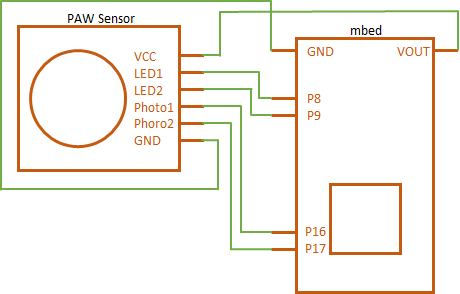# PAW Sensor

Tactile sensor that can detect the amount of displacement.

## Hello World

#### Import programPAW_Sensor_HelloWorld

This program is example of using PAW_Sensor Library.

## Library

#### Import libraryPAW_Sensor

PAW_Sensor: This library control the PAW sensor. This library get sensor values from the PAW sensor, and send these values to PC through serial-communication. And you need to convert these values to delta h using some module on PC. I create sample python module for this library. If you want to get this module, please access below page, https://github.com/HiroakiMatsuda/PAW-Sensor The PAW Sensor is developed by RT. If you need to get information about this sensors, please access below page. http://www.rt-shop.jp/index.php?main_page=product_info&cPath=42&products_id=1303

## Pinout## Notes

Data Format

PAW_Sensor Library send values through a serial-communication. This data-format is as below.

• ID: 0 ~ 255. This value is used to identify a plurality of PAW Sensors.
• ChN low: Lower bits of the Voltage of each channel.
• ChN high: Upper bits of the Voltage of each channel.

How to decode this data

ch_n = ( ( ch_n_high << 8 ) & 0xFF00 ) | ( ch_n_low & 0xFF )

• ch_n_low: Lower bits of the Voltage of each channel.
• ch_n_high: Upper bits of the Voltage of each channel.
• ch_n: Decoded Voltage of each channel.

How to calculate dH

dh_ch_n = cna * ( ch_n - ch_n_init ) ^4 + cnb * ( ch_n - ch_n_init )^3 + cnc * ( ch_n - ch_n_init )^2 + cnd * ( ch_n - ch_n_init )

• ch_n: Decoded Voltage of each channel.
• ch_n_init: Voltage at no load of ch_n
• cna: The coefficient of specific to the PAW Sensor
• cnb: The coefficient of specific to the PAW Sensor
• cnc: The coefficient of specific to the PAW Sensor
• cnd: The coefficient of specific to the PAW Sensor

Sample python module for PAW_Sensor Library

You need to parse data from mbed through a serial-communication. If you want to know how to parse this data, please access below page,

https://github.com/HiroakiMatsuda/PAW-Sensor